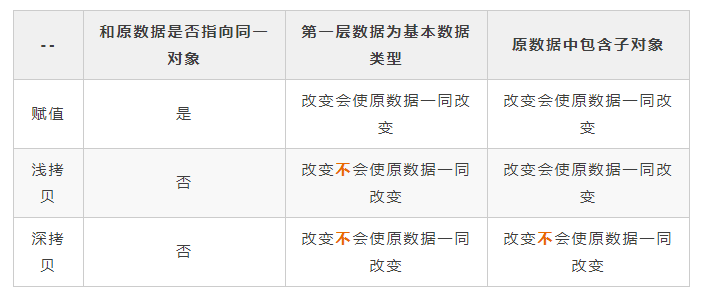doclist 阅读(62) 评论(0)

### 参考:

#### JavaScript 数据类型

b = a 就是一次拷贝，重新给 b 赋值，a 的值不会改变：

```const a = 5
let b = a // this is the copy

b = 6

console.log(b) // 6
console.log(a) // 5```

```const a = {
en: 'Hello',
de: 'Hallo',
es: 'Hola',
pt: 'Olà'
}
let b = a
b.pt = 'Oi'
console.log(b.pt) // Oi
console.log(a.pt) // Oi```

```const a = {
en: 'Bye',
de: 'Tschüss'
}
let b = {...a}
b.de = 'Ciao'
console.log(b.de) // Ciao
console.log(a.de) // Tschüss```

Object.assign()

```const a = {
en: 'Bye',
de: 'Tschüss'
}
let b = Object.assign({}, a)
b.de = 'Ciao'
console.log(b.de) // Ciao
console.log(a.de) // Tschüss```

```const a = [1,2,3]
let b = [...a]
b = 4
console.log(b) // 4
console.log(a) // 2```

```const a = [1,2,3]
let b = a.map(el => el)
b = 4
console.log(b) // 4
console.log(a) // 2```

```const a = [1,2,3]
const b = a.map((el, index) => index === 1 ? 4 : el)
console.log(b) // 4
console.log(a) // 2```

Array.slice

```const a = [1,2,3]
let b = a.slice(0)
b = 4
console.log(b) // 4
console.log(a) // 2```

```const a = {
foods: {
dinner: 'Pasta'
}
}
let b = {...a}
b.foods.dinner = 'Soup' // changes for both objects
console.log(b.foods.dinner) // Soup
console.log(a.foods.dinner) // Soup```

## 深拷贝

```const a = {
foods: {
dinner: 'Pasta'
}
}
let b = {foods: {...a.foods}}
b.foods.dinner = 'Soup'
console.log(b.foods.dinner) // Soup
console.log(a.foods.dinner) // Pasta```

`const b = {...a, foods: {...a.foods}}.`

```const a = {
foods: {
dinner: 'Pasta'
}
}
let b = JSON.parse(JSON.stringify(a))
b.foods.dinner = 'Soup'
console.log(b.foods.dinner) // Soup
console.log(a.foods.dinner) // Pasta```

1. 会忽略 undefined
2. 会忽略 symbol
3. 不能序列化函数
4. 不能解决循环引用的对象
```// 木易杨
let obj = {
name: 'muyiy',
a: undefined,
b: Symbol('muyiy'),
c: function() {}
}
console.log(obj);
// {
//     name: "muyiy",
//     a: undefined,
//  b: Symbol(muyiy),
//  c: ƒ ()
// }

let b = JSON.parse(JSON.stringify(obj));
console.log(b);
// {name: "muyiy"}```

```// 木易杨
let obj = {
a: 1,
b: {
c: 2,
d: 3
}
}
obj.a = obj.b;
obj.b.c = obj.a;

let b = JSON.parse(JSON.stringify(obj));
// Uncaught TypeError: Converting circular structure to JSON```

## 拷贝自定义类型的实例

```class Counter {
constructor() {
this.count = 5
}
copy() {
const copy = new Counter()
copy.count = this.count
return copy
}
}
const originalCounter = new Counter()
const copiedCounter = originalCounter.copy()
console.log(originalCounter.count) // 5
console.log(copiedCounter.count) // 5
copiedCounter.count = 7
console.log(originalCounter.count) // 5
console.log(copiedCounter.count) // 7```

如果实例中有其它对象的引用，就要在copy方法中使用  JSON.stringify 和 JSON.parse 。

除此之外，深拷贝方法还有 jQuery.extend() 和 lodash.cloneDeep()

## 总结：